# [Solved] Write the Following as Decimals. (See.)

Question 48
Essay

## Write the following as decimals. (See.) Writing Fractions as PercentsA marketing manager is given the following data in fraction form and must change the data to percents.(a)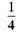(b)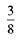(c)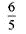SOLUTION First write each fraction as a decimal, and then write the decimal as a percent.(a)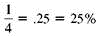(b)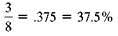(c)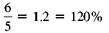Writing Percents as Decimals To calculate some insurance claims, an insurance agent must change the following percents to decimals.(a) 35%(b) 50%(c) 325%(d)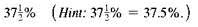Quick.TIPChange any fraction part of a percent to a decimal before converting from a percent to a decimal.SOLUTION Move the decimal point two places to the left and drop the percent sign.(a).35(b).5(c) 3.25(d).375Writing Percents as Fractions Even though smoking is believed to account for 1 of every 5 deaths in the United States, more than 45 million Americans smoke. The bar chart shows the percent of people in each age group that smoke. Convert each percent to a fraction and reduce to lowest terms.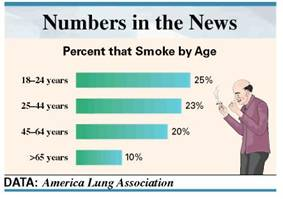SOLUTION Write each percent as a decimal and then as a fraction in lowest terms.(a)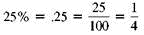(b)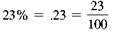(c)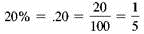(d)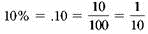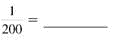10+ million students use Quizplus to study and prepare for their homework, quizzes and exams through 20m+ questions in 300k quizzes.

Explore our library and get Management Homework Help with various study sets and a huge amount of quizzes and questions

3.5K

Study sets

66.5K

Quizzes

5.9M

Questions

Upload material to get free accessInvite a friend and get free accessSubscribe and get an instant access